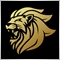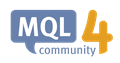# OrderOpenPrice question .173
husonchows

I have no idea why my OrderOpenPrice() would remember the last price even in Backtesting with different pair .

for example , last OrderOpenPrice was eurusd 1.00000 , and I test on usdjpy , the OrderOpenprice will be 1.00000 also . and usdjpy was no order begin.Moderator
12762

You have to show the code.

Did you select the order by using OrderSelect() function?

# OrderOpenPrice

Returns open price of the currently selected order.

 double  OrderOpenPrice();

Returned value

Open price of the currently selected order.

Note

The order must be previously selected by the OrderSelect() function.

Example:

 ```  if(OrderSelect(10, SELECT_BY_POS)==true)     Print("open price for the order 10 ",OrderOpenPrice());   else     Print("OrderSelect returned the error of ",GetLastError());```OrderSelect - Trade Functions - MQL4 Reference
• docs.mql4.com
OrderSelect - Trade Functions - MQL4 Reference173
husonchows
```

//Breakeven Price
double be_JG_duod(double &be_JG_kongd){
double jg_duod=0;
double jg_kongd=0;
double xz_kcJG=OrderOpenPrice();
double ot=OrdersTotal();
for(int k=0;k<ot;k++){
if(OrderSymbol()==Symbol()&& OrderMagicNumber()==hm_1 && OrderComment()==zs_1){
if(OrderType()==kc_lx_0){
jg_duod+=syg_kcJG;
}
if(OrderType()==kc_lx_1){
jg_kongd+=syg_kcJG;
}
}
}
}
be_JG_kongd=jg_kongd;
return(jg_duod);
}

//Breakeven Lots
double be_SS_duod(double &be_SS_kongd){
double ss_duod=0;
double ss_kongd=0;
double DL=OrderLots();
double ot=OrdersTotal();
for(int j=0;j<ot;j++){
if(OrderSymbol()==Symbol()&& OrderMagicNumber()==hm_1 && OrderComment()==zs_1){
if(OrderType()==kc_lx_0){
ss_duod++;
}
if(OrderType()==kc_lx_1){
ss_kongd++;
}
}
}
}

be_SS_kongd=ss_kongd;
return(ss_duod);
}

double BE_DUOD(double &BE_KONGD){
double be_JG_kongd;
double be_JG_duod=(double)be_JG_duod(be_JG_kongd);
double be_SS_kongd;
double be_SS_duod=(double)be_SS_duod(be_SS_kongd);
double beduod=0;//beduod=NormalizeDouble(beduod,5);
double bekongd=0;//bekongd=NormalizeDouble(bekongd,5);
double ot=OrdersTotal();
for(int j=0;j<ot;j++){
if(OrderSymbol()==Symbol()&& OrderMagicNumber()==hm_1 && OrderComment()==zs_1){
if(OrderType()==kc_lx_0){
if(be_JG_duod!=0 || be_SS_duod!=0){
beduod=be_JG_duod/be_SS_duod; Print("be_JG_duod="+be_JG_duod+"be_SS_duod+"+be_SS_duod);
}
}
if(OrderType()==kc_lx_1){
if(be_JG_kongd!=0 || be_SS_kongd!=0){
bekongd=be_JG_kongd/be_SS_kongd;
}
}
}
}
}
BE_KONGD=bekongd=NormalizeDouble(bekongd,5);
return(beduod);
}

```173
husonchows
Tsz Ho Chow:

Actually , I want to get the breakeven price , but my breakeven price always wrong , and finally , if I try to print the OrderOpenPrice() in OnTick() , it will show the last OrderOpenPrice even it's from different pair.

and I have tried to correct the code , and it's still wrong for the breakeven ....need help . The Breakeven will become the price of last order . dont know why .20368

Tsz Ho Chow:Actually , I want to get the breakeven price , but my breakeven price always wrong
 Break even price is lots weighted average price. Sum( lots[i] * OOP[i] ) / Sum( lots[i] ) 1@6 + 2@10 = 26/3 = 8.667 = 1@(+2.667) + 2@(-1.333) = 0.001 If you have hedging trades include their direction (dir buy=+1, sell=-1) thus: Sum( dir(i) * lots[i] * OOP[i] ) / Sum( dir(i) * lots[i] ) 1@6 - 2@10 = -14/-1 = 14.00 = 1@(+8)-2@(-4) = 0173
husonchows
whroeder1:
 Break even price is lots weighted average price. Sum( lots[i]*OOP[i] ) / Sum(lots[i])

Actually , I am trying to get the simple average price . still can not . lol ( up there , I try to get "Lots total " and " Orders total" and Orders total / orders total . finally the average price is incorrect too ..i need to think about it . i have to do this .20368

 Tsz Ho Chow:  I am trying to get the simple average price . Lots weighted average price, is the break even price, is the only average that makes sense.20368

 Tsz Ho Chow: , I have searched something about " Bars " it's uesless on demo/live account .```bool sm_kx(){ static int yiyou_kx=0; if(Bars==yiyou_kx) return(false); yiyou_kx=Bars; return(true); } ``` Nothing wrong with Bars (on either.) Your use of it is.For a new bar test, Bars is unreliable (a refresh/reconnect can change number of bars on chart,) volume is unreliable (miss ticks,) Price is unreliable (duplicate prices and The == operand. - MQL4 forum.) Always use time. New candle - MQL4 forum I disagree with making a new bar function, because it can only be called once per tick. A variable can be tested multiple times.Moderator
12762

```//Breakeven Price
double be_JG_duod(double &be_JG_kongd){
double jg_duod=0;
double jg_kongd=0;
double xz_kcJG=OrderOpenPrice();
double ot=OrdersTotal();
for(int k=0;k<ot;k++){

Here you call OrderOpenPrice() function, but there is no order selected (yet).

You can only call this function after an order has been selected by OrderSelect() function.

```//Breakeven Lots
double be_SS_duod(double &be_SS_kongd){
double ss_duod=0;
double ss_kongd=0;
double DL=OrderLots();
double ot=OrdersTotal();
for(int j=0;j<ot;j++){

And here you make the same mistake, but this time you call OrderLots() function, before and order has been selected by OrderSelect() function.173
husonchows
Marco vd Heijden:

Here you call OrderOpenPrice() function, but there is no order selected (yet).

You can only call this function after an order has been selected by OrderSelect() function.

And here you make the same mistake, but this time you call OrderLots() function, before and order has been selected by OrderSelect() function.

Thanks for your help . Do you mean i should make it like this ? but the same problem occur to me , the average price become the last OrderOpenPrice . lol
```double be_SS_duod(double &be_SS_kongd){
double ss_duod=0;
double ss_kongd=0;
double ot=OrdersTotal();
for(int j=0;j<ot;j++){
if(OrderSymbol()==Symbol()&& OrderMagicNumber()==hm_1 && OrderComment()==zs_1){
double DL=OrderLots();
if(OrderType()==kc_lx_0){
ss_duod++;
}
if(OrderType()==kc_lx_1){
ss_kongd++;
}
}
}
}

be_SS_kongd=ss_kongd;
return(ss_duod);
}```Moderator
12762